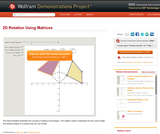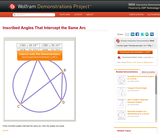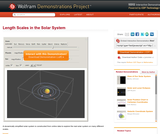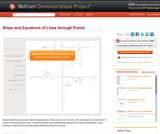Updating search results...

# 4 Results

View
Selected filters:
• Wolfram Demonstrations ProjectConditional Remix & Share Permitted
CC BY-NC-SA
Rating
5.0 stars

This Demonstration illustrates the concept of rotating a 2D polygon. The rotation matrix is displayed for the current angle. The default polygon is a square that you can modify.

Subject:
Geometry
Material Type:
Activity/Lab
Diagram/Illustration
Homework/Assignment
Interactive
Simulation
Teaching/Learning Strategy
Provider:
Wolfram Research
Provider Set:
Wolfram Demonstrations Project
Author:
Mito Are and Valeria Antohe
09/04/2013Conditional Remix & Share Permitted
CC BY-NC-SA
Rating
0.0 stars

If two inscribed angles intercept the same arc, then the angles are equal. Drag the orange points to change the figure.

Subject:
Geometry
Material Type:
Activity/Lab
Diagram/Illustration
Homework/Assignment
Interactive
Simulation
Teaching/Learning Strategy
Provider:
Wolfram Research
Provider Set:
Wolfram Demonstrations Project
Author:
Jay Warendorff
09/04/2013Conditional Remix & Share Permitted
CC BY-NC-SA
Rating
0.0 stars

A dynamically simplified solar system is constructed from online data to explore the real solar system on many different scales.

The realistically scaled solar system is surprising because nothing is visible due to the presence of many different scales. That is why it is usually rescaled in animations or illustrations. This is nice but gives us a wrong sense of distances and sizes. This Demonstration is intended to show the solar system's different scales in their full glory.

Since it is hardly possible to see anything when the real scales are used, controls have been added to modify the sizes of the celestial bodies.

Subject:
Astronomy
Material Type:
Activity/Lab
Diagram/Illustration
Homework/Assignment
Interactive
Simulation
Teaching/Learning Strategy
Provider:
Wolfram Research
Provider Set:
Wolfram Demonstrations Project
Author:
Cedric Voisin
09/04/2013Conditional Remix & Share Permitted
CC BY-NC-SA
Rating
0.0 stars

Algebra students need practice determining equations of lines given a pair of points, or the line parallel or perpendicular to a given line through a given point. This Demonstration, along with guiding worksheets or a teacher presentation, gives students a chance to see the relationships between these lines and points.

Subject:
Algebra
Material Type:
Activity/Lab
Diagram/Illustration
Homework/Assignment
Interactive
Simulation
Teaching/Learning Strategy
Provider:
Wolfram Research
Provider Set:
Wolfram Demonstrations Project
Author:
Tom Falcone